# Simplify Linear Expressions

Simplifying an expression is a key skill in algebra.

We use algebra to solve numerical problems and to make formulas.

When we play a game we can make two kinds of move: a defensive move or an offensive move. In algebra there are two types of move: a simplifying move or a balancing move.

simplifying move is when you alter one both sides of your equations – the result is that the side looks different but has the same value. For example:The left hand side simplifies toso we can write:balancing move is when perform the same operation to both sides to keep the equation balanced. For example:## Numbers as Letters

In algebra we represent a number with a single letter.

Here’s an interesting article on math notation that puts math language in its place as a human invention with the intention of making math life more simple.

We use letters for two reasons:

1. We know the meaning of the number, but we don’t know the value (unknown).

Eg, the number of gift cards I will sell at the craft fair tomorrow. Suppose I sell a single gift card for 4 dollars. Lets chose the letterfor the number of cards I end up selling. I will makedollars, which we can write compressed as.

2. We know the meaning of the number and the number changes (variable).

Eg, My heartrate during a workout at the gym. I know that my heartrate will be between 50 and180 beats per minute (bpm). Let’s choose the letterfor this number. My heartratewill vary between 50 bpm and 180 bpm during the workout.

## Definitions

A term is a single number or unknown (a number represented by a letter), or the product (multiplication) of a number with unknowns. If the unknowns become known, the value of the term can be calculated. Terms can be positive or negative.

Here are four terms:Suppose. Then the value of the termis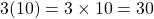.

An expression is the addition of one or more terms. If the unknowns become known the value of the expression can be calculated.

Here is an expression made by adding four terms together (note that adding a negative is the same as subtracting):This expression has two unknowns, represented byand.

Writing two unknowns together means to multiply them, egmeans.

If it is given thatand, the value of this expression is:Note that this expression can be written in any order because addition is commutative:A linear expression is an expression that includes a constant or an unknown or both. The unknown has power one. When we draw a graph of a linear expression (linear relation), the graph is a straight line. This is why it is called ‘linear’.

Here are four linear expressions:.

# Simplify An Expression

We simplify an expression when we collect like terms together. This is kind of like organising the cutlery drawer, or counting a jar full of coins.https://farm2.static.flickr.com/1431/566441814_6004fe8712_b.jpg

When sorting the coins, we generally sort by the type of coin. E.g. put all the loonies together, the toonies together etc to simplify the final calculation.

What counts as ‘simple’ can be debated. It really depends on the context. The skills to have to hand are the following:

• gather like terms;
• expand brackets;
• factor a common factor.

## Gather like terms

We gather like terms to reduce the number of terms an expression has. This is just like combining all the forks together and all the knives together, except that we are gathering like numbers.

Example 1:.

Note that when a letter appears without a coefficient, the coefficient is 1.

Example 2:.

When we have two or more different letters or different combinations of letters, we remember that different letters stand for different numbers. We can’t gather different letters together.

Example 3: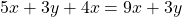.

Constants state their own value. We can gather constants together.

Example 4: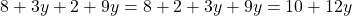.

Number combinations need to stick together:

Example 5:Try it out here:

applet

## Expand brackets

We use brackets (parenthesis) when we need to multiply a whole expression by a number or by another expression.

Let’s take the number, and multiply the whole thing by.

If we simply sayit looks like we are multiplying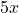by 3 and poor 4 is left behind. That’s why we need brackets.

Remember that multiplication is shorthand for repeated addition so let’s decode:.

The distributive property lets us do this more efficiently:

The distributive property states that.

In other words,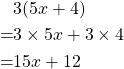Try it out here:

applet

## Factor

To factor a linear expression is to reverse the ‘expand the brackets’ process. To do it we look for a common factor in each term.

We say ‘factor fully’ to make sure we take the greatest common factor from each terms.Try it out here:

applet

## Keep the Value: a checking mechanism

The value of an expression before any simplifying process must be the same as the value after the process. We can check that our simplification is correct by testing a random number in the before version and in the after version.

Consider the expression.

Suppose,.

Now, lets factor the expression.Now, leton the new expression,.  We have,as before.

The before is equal to the after, and so its very likely that our factoring is accurate.

## Expand, Simplify, Factor

Simplifying is an important part of handling algebra. To simplify an expression makes  our math more efficient and reduces the possibilities for errors.

Check out the old equations on this page. Solving equations becomes more straightforward when we simplify both sides before beginning the solving process. Remember that simplifying changes how an expression appears (looks) but not its value.